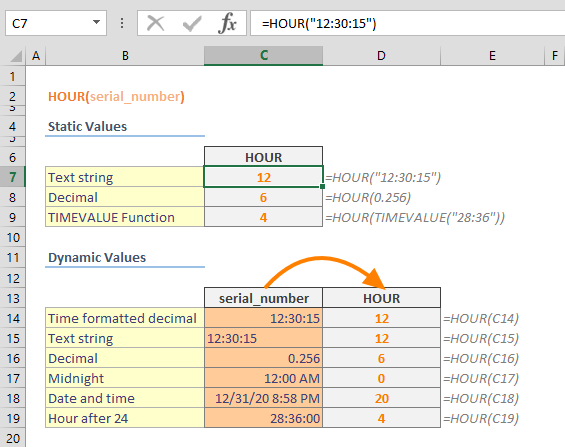The Excel Hour function is a Date & Time formula that parses and returns the hour value from a time value. The return value is an integer, ranging from 0 (12:00 A.M.) to 23 (11:00 P.M.). In this guide, we’re going to show you how to use the Excel HOUR function and also go over some tips and error handling methods.

## Supported versions

• All Excel versions

## Excel HOUR Function Syntax

HOUR(serial_number)

## Arguments

 serial_number The time value that contains the hours you want to find. You can use a text string like "8:30" or a decimal number like 0.24125 (in Excel this value means 5:47:24 AM).

## Excel HOUR Function Examples

The Excel HOUR function only needs single argument to execute, serial_number. Although the argument name contains "number" in its name, Excel can evaluate time values in strings as well. Simply enter the serial_number as a static value, a cell reference, or a return value from a function that can able to generate a time value. Functions like TIME and TIMEVALUE can be used for this purpose.

=HOUR("12:30:15") returns 12

=HOUR(0.256) returns 6

=HOUR(TIMEVALUE("28:36")) returns 4 because HOUR resets time after 24.## Tips

• Excel stores date and time as numbers. Excel assumes that the date 1/1/1900 is “1″, and increases this number by 1 for each subsequent day. As a result of this, the time values would have to be between 0 and 999999999. For example; 6:00 AM is equal to 0.25. Because of this, the HOUR function in Excel returns a number that is displayed as a time value.
• VBA also has a function that shares the same name. The use case for both functions are very similar.
``` Dim MyTime, MyHour
MyTime = #4:35:17 PM#    ' Assign a time.
MyHour = Hour(MyTime)    ' MyHour contains 16.

You can use MINUTE and SECOND functions to get minute and second values from a time value respectively.

Issues

The HOUR function returns a #NUM! error, if the serial_number entered is negative or greater than the limit of 999999999.
```